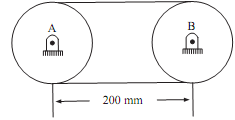## Find out the largest torque, C/C++ Programming

Assignment Help:

Find out the largest torque:

A flat belt is taken in use to transmit torque from pulley A to pulley B as shown in figure given below. The radius of each pulley is 50mm and coefficient of friction is 0.3. Find out the largest torque that can be transmitted if the allowable belt tension is 3KN.

Sol: Radius of each pulley = 50mm, R1 = R2  = 50mm

R1 = Radius of driver = 50mm

R2 = Radius of driven = 50mm

θ = Angle of contact= 1800 = p,

µ = Coefficient of friction = 0.3T1 = Allowable tension = 3KN

T1  greater than T2

Using relation T1/T2  = eµθ

By putting all the value,

3/T2  = e(0.3)(p)

On solving T2  = 1.169KN                                                                       .......ANS

Since Radius of both pulley is same;

So, Torque exerted on both the pulley is same and

= (T1-T2).R1  = (T1-T2).R2

By putting all the value we get,

(3 - 1.169) X 50 = 91.55 KN-mm                                                    .......ANS

#### Write code to implement constructor and assessors, Study the given class sp...

Study the given class specification and answer the questions that follow: class Person { private:   string name;   int yrBorn;   int yrDied;   public:   Per

what is oop?

#### C/c++ program, luminous jewel polishing necklace,c/c++ programming

luminous jewel polishing necklace,c/c++ programming

#### Compute the average of five numbers, Step 1 Define the program headers and ...

Step 1 Define the program headers and the variables      #include   #include   #include   #include void main()   {       char prompt;     float a,b,c,d,e;     floa

#### Write a recursive function with cylinders, I need to make a fractal trees i...

I need to make a fractal trees in OpenGL C++ . The method used is to write a recursive function with cylinders of different sizes and angles to create the tree trunk and branches.

#### Assignment, electricity biil code

electricity biil code

#### Define the bitwise-exclusive-or operator, Define the Bitwise-Exclusive-OR O...

Define the Bitwise-Exclusive-OR Operator: ^: The bitwise-exclusive-OR operator (^) compares every bit of its first operand to the corresponding bit of its second operand. If on

#### Plugging the memory leak, Problem #1: plugging the memory leak. When you em...

Problem #1: plugging the memory leak. When you employ the "normal" new operator, for example Foo* p = new Foo(), the compiler generates some special code to manage the case while t

#### #decode the code, #Smugglers are becoming very smart day by day. Now they h...

#Smugglers are becoming very smart day by day. Now they have developed a new technique of sending their messages from one smuggler to another. In their new techn

#### Program is to append the contents of one file to another, Program is to app...

Program is to append the contents of one file to another: void main()    {   clrscr();   fstream file1,file2;   char st1,st2;/* 13 because a filename canno